Impact Factor 4.566 | CiteScore 5.6
More on impact ›

# Frontiers in Physiology

## ORIGINAL RESEARCH article

Front. Physiol., 19 July 2010 | https://doi.org/10.3389/fphys.2010.00022

# Bistable behavior of the lac operon in E. coli when induced with a mixture of lactose and TMG

• 1 Escuela Superior de Física y Matemáticas, Instituto Politécnico Nacional, Mexico D.F., Mexico
• 2 Unidad Monterrey, Centro de Investigación y de Estudios Avanzados del IPN, Apodaca, Nuevo León, Mexico
• 3 Centre for Applied Mathematics in Bioscience and Medicine, McGill University, Montreal, QC, Canada

In this work we investigate multistability in the lac operon of Escherichia coli when it is induced by a mixture of lactose and the non-metabolizable thiomethyl galactoside (TMG). In accordance with previously published experimental results and computer simulations, our simulations predict that: (1) when the system is induced by TMG, the system shows a discernible bistable behavior while, (2) when the system is induced by lactose, bistability does not disappear but excessively high concentrations of lactose would be required to observe it. Finally, our simulation results predict that when a mixture of lactose and TMG is used, the bistability region in the extracellular glucose concentration vs. extracellular lactose concentration parameter space changes in such a way that the model predictions regarding bistability could be tested experimentally. These experiments could help to solve a recent controversy regarding the existence of bistability in the lac operon under natural conditions.

## Introduction

Mathematical models and computer simulations of genetic networks have demonstrated collective behaviors (commonly called systems, or emergent properties) that were not apparent from examination of the network components. Among the various patterns of complex behavior associated with non-linear kinetics, multistability is noteworthy. A system is said to be multistable when it can rest in two or more stable steady states for a given parameter set. By smoothly changing the parameter values one can make the stable steady state count discontinuously change, via saddle-node bifurcations. This allows a graded signal to be turned into a discontinuous evolution of the system (usually accompanied by hysteresis) along different discrete states.

Multistability has been invoked to explain catastrophic events in ecology (Rietkerk et al., 2004), mitogen-activated protein kinase (MAPK) cascades in animal cells (Ferrell Jr. and Machleder, 1998; Bagowski and Ferrell, 2001; Bhalla et al., 2002), cell cycle regulatory circuits in Xenopus and Saccharomyces cerevisiae (Cross et al., 2002; Pomerening et al., 2003), the generation of switch-like biochemical responses (Ferrell Jr. and Machleder, 1998; Bagowski and Ferrell, 2001; Bagowski et al., 2003), and the establishment of cell cycle oscillations and mutually exclusive cell cycle phases (Pomerening et al., 2003; Sha et al., 2003), among other biological phenomena.

The two paradigmatic gene-regulatory networks concerning bistability are the phage λ switch and the lac operon of Escherichia coli (Chung and Stephanopoulos, 1996; Ferrell, 2002; Angeli et al., 2004). As a matter of fact, bistability in biochemical networks was first discovered in the lac operon of E. coli, when induced with non-metabolizable inducers (Novick and Weiner, 1957; Cohn and Horibata, 1959). In the phage λ switch, bistability arises through a mutually inhibitory double-negative-feedback loop, and it is responsible for the decision between the lytic and the lysogenic pathways made by bacteria after being infected by the phage λ virus. In the lac operon, a positive-feedback loop is responsible for bistability, and this phenomenon has been adduced to explain how E. coli feeds on lactose but only when glucose (its favorite carbon source) is absent from the growing medium.

Recently, Ozbudak et al. (2004) quantified the lac operon bistable behavior by carrying out a series of clever experiments with genetically modified E. coli bacteria. Their results confirmed the existence of bistability in this system, when it is induced by the non-metabolizable inducer thiomethyl galactoside (TMG), but failed to demonstrate bistability when the operon was induced with lactose.

The results of Ozbudak et al. (2004) have open a controversy regarding the existence of bistability in the lac operon of E. coli under natural conditions. On the one hand, van Hoek and Hogeweg (2006) carried out in silico simulation of the lac operon evolution in bacterial populations. In these simulations, the parameters that control the expression of the lac operon genes evolve in such a way that the system is not bistable when the bacterial population grows in a lactose rich medium, but it does exhibit bistability when artificial inducers are employed. Thus, van Hoek and Hogeweg (2006) argue from their computational experiments that the wild-type lac operon is not a bistable switch under natural conditions. On the other hand, Santillán and colleagues (Santillán et al., 2007; Santillán, 2008) developed a detailed mathematical model for the lac operon regulatory pathway (estimating the model parameters from reported experimental data) and examined the effect of lactose metabolism. According to their results, lactose metabolism greatly modifies the system bistable region, in the external lactose vs. external glucose parameter space, but does not make this behavior disappear.

The current understanding of the lac operon alludes to bistability to explain how E. coli consumes glucose and lactose in the most efficient possible way (Lehninger et al., 2005). Namely, the genes necessary to uptake and metabolize lactose are only turned on when this sugar is present (at high levels) in the growing environment, while glucose (the bacterium favorite carbon source) is absent. If, as some studies suggest, bistability is lacking in the lac operon under natural conditions, this would open the question of how the regulatory pathway in this system allows an efficient consumption of alternative carbon sources.

Interestingly, the gene networks responsible for the alternate consumption of glucose and tryptophan in E. coli (Konan and Yanofsky, 1997), and of glucose and galactose in S. cerevisiae (Acar et al., 2005; Bhat and Iyer, 2009), have the same architecture as the lac operon of E. coli. That is, the regulatory pathway controlling the expression of the genes involved in the transport and metabolism of carbon sources other than glucose seems to be conserved over different metabolic networks and over different species. We see from these facts that the issue of whether the lac operon is bistable or not in its natural habitat transcends this system, and the clarification of this question may help to understand the performance of a whole class of gene networks. Moreover, experiments similar to the ones here proposed may help to study bistability in other gene networks that allow the cell to transport and metabolize carbon sources other than glucose.

As mentioned above, the modeling studies of Santillán and colleagues (Santillán et al., 2007; Santillán, 2008) predict that the lac operon shows bistability when induced with lactose. Unfortunately, as asserted by the same authors, is quite hard to experimentally test this prediction. With this in mind, the purpose of the present work is to extend the Santillán et al. (2007) model (by considering the operon induction with a mixture of lactose and TMG) in order to propose feasible experiments whose results will validate or disprove it, as well as its predictions regarding bistability. Similar experiments may help to elucidate whether bistability exists or not in other gene networks with the same architecture. This work is in the line of Acar et al. (2005), who suggested methods for experimentally studying bistability in S. cerevisiae.

## Materials and Methods

### The Lactose Operon Regulatory Pathway

The genes in the lac operon code for the proteins necessary to transport and metabolize lactose. These genes, which span a DNA segment around 6000 bp long, are: lacZ, lacY, and lacA. The protein coded by lacZ forms an homotetrametic enzyme called β-galactosidase. This enzyme metabolizes lactose into either allolactose (approximately half of it) or into glucose and galactose (the other half). The same enzyme is also responsible for metabolizing allolactose into glucose and galactose. On the other hand, lacY codes for a permease involved in the uptake of lactose from the environment, while lacA codes for thiogalactoside transacetylase, which plays no role in the operon regulatory pathway. Lactose permease is also capable of transporting gratuitous inducers (like TMG or IPTG), but they cannot be metabolized by β-galactosidase.

The elements involved in the lac operon regulation are located as shown in Figure 1A. There, P represent the promoter, C is the binding site for the catabolite activator protein (which enhances transcription initiation), and O1, O2, and O3 stand for active repressor protein binding sites (Oehler et al., 1990).

FIGURE 1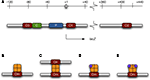Figure 1. (A) Schematic representation of the regulatory elements located in lac operon DNA. P denotes the promoter, O1, O2, and O3 correspond to the three operators (repressor binding sites), and C is the binding site for the cAMP–CRP complex. The different ways in which a repressor molecule can interact with the operator sites are represented in (B–E). Namely: a free repressor molecule (B), one with a single subunit bound by an inducer molecule (D), or one with the two subunits in the same side bound by allolactose (E) can bind a single operator. Moreover, a free repressor molecule can bind two different operators simultaneously (C). Figure adapted from Santillán (2008).

Glucose is the favorite carbon and energy source for E. coli, as well as for many other organisms. Although this bacterium can also feed on other sugars (like lactose), it only does so when glucose is absent. Thus, if a bacterial culture grows in a medium containing a mixture of glucose and another sugar, it will exclusively feed on the former until it is exhausted, and only then will it switch to the second one. A consequence of this behavior is that the bacterial growth curve shows two distinctive phases. This phenomenon was originally studied by Monod (1941), who described it as diauxic growth. As seen below, the regulatory mechanisms in this operon are responsible for diauxic growth in E. coli. The regulatory mechanisms responsible for this behavior are reviewed below. We refer the reader to the schemes in Figure 2 for a better understanding.

FIGURE 2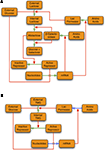Figure 2. Schemes representing the lac operon regulatory pathways when the system is induced with lactose (A), and when it is induced with an artificial inducer like TMG (B). Labeled rectangles represent chemical species, arrows with empty heads denote processes through which one chemical species is transformed into another, arrows with solid heads indicate interactions that enhance the process they point to, and finally, lines ending in perpendicular bars denote interactions that diminish the process they point to.

When glucose is absent from the bacterial growing medium, cyclic AMP (cAMP) is synthesized at high rates. In turn, cAMP binds CRP proteins turning them active. Finally, active CRP proteins (also known as catabolite activator proteins, CAP) bind an specific site right upstream of promoter lacP and considerably increase its affinity for mRNA polymerase. On the other hand, the presence of glucose in the medium prevents the enhancement of transcription initiation because cAMP is not synthesized, and thus CRP proteins are not activated. This regulatory mechanism is known as catabolite repression.

Another regulatory mechanism operates through transcriptional repression and involves a repressor molecule. The lac repressor is a homo-tetramer (consisting of two functional homo-dimers) of LacI polypeptides (Lewis, 2005; Wilson et al., 2007). Each functional dimer can bind Operators O1, O2, and O3. Whenever a repressor is bound to operator O1, it avoids transcription initiation by blocking the mRNA polymerase. On the other hand, DNA can fold in such a way that a single repressor binds two operators simultaneously, one per dimer. The effect of these loops is to enhance the stability of the corresponding complex.

Each monomer in the lac repressor can be bound by an allolactose or a TMG molecule, inhibiting the capability of the corresponding dimer to bind an operator. This means that free repressors can bind one operator (Figure 1B), or two of them simultaneously (Figure 1C); that repressors with three free monomers can bind one but not two operators (Figure 1D); that repressors with two free monomers can bind one operator, if the bound monomers belong to the same dimer (Figure 1E), or none at all; and that repressors with only one free monomer, as well as repressors with all four monomers bound by an inducer molecule (Narang, 2007), are unable to bind any operator. Therefore, active repressors are inhibited, and so repression is relieved, by increasing the intracellular inducer concentration.

The last regulatory mechanism in the lac operon is the so-called inducer exclusion. In it, external glucose decreases the efficiency of lac permease to transport lactose (Reznikoff, 1992), and by doing so negatively affects the induction of the operon genes.

### Model Development

In Section “Model Development” in Appendix we develop a mathematical model for the lac operon regulatory pathway when it is induced with a mixture of lactose and the artificial inducer TMG. This model is based on a previous model introduced by Santillán (2008), which is modified to take into account the presence of TMG. Like the original model, the present one accounts for all of the regulatory mechanisms described in the previous subsection.

The model here introduced consists of four ordinary differential equations that account for the dynamics of mRNA concentration [M], LacE polypeptide concentration [E], intracellular lactose concentration [L], and intracellular TMG concentration [T]. The model equations are given in Table 1 and are briefly described in the forthcoming paragraphs. Equation (1) accounts for the mRNA concentration dynamics. There, kM stands for the transcription initiation rate of the active lacP promoter; ppc([Ge]) is a function of the extracellular glucose concentration ([Ge]) – defined in (9) – is the probability that a mRNA polymerase is bound to promoter lacP, taking into account cooperativity with a CAP protein bound to its corresponding site; function 𝒫R([A],[T]) – defined in (12) – is the probability that the lac promoter is not repressed and so that a polymerase bound to it can initiate transcription; [A] is defined in (5) and represents the intracellular allolactose concentration; parameter γM represents the mRNA degradation rate; and μ corresponds to the bacterial growth rate. In this work we consider a variable growth rate, which depends on the glucose uptake flux (20) and on the lactose metabolism rate (21) as given by Equation (8); see Santillán (2008) for a derivation of this equation.

Equation (2) stands for the LacE polypeptide concentration dynamics. The meaning of parameters in it is as follows: kE is the translation initiation rate at the ribosome binding site of gene lacE and γE is the degradation rate of lacE polypeptides.

TABLE 1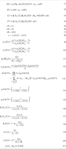Table 1. Mathematical model for the lac operon regulatory pathway in E. coli, which accounts for the operon simultaneous induction with lactose and TMG.

Equation (3) governs the intracellular lactose concentration dynamics. There, kL is the maximal lactose uptake rate per permease molecule; [Q] – defined in (6) – is the concentration of permease molecules; β([Le],[Te]) represents permease activity for lactose transport as a function of the extracellular lactose ([Le]) and TMG ([Te]) concentrations; βG([Ge]) accounts for the inducer exclusion regulatory mechanism, which is known to depend on [Ge]; φM is the maximal lactose metabolism rate per β-galactosidase; [B] – defined in (7) – denotes β-galactosidase concentration; and ℳ ([L]) – defined in (19) – corresponds to the activity of β-galactosidase enzymes, as a function of intracellular lactose concentration.

Analogously, Equation (4) governs the dynamics of the intracellular TMG concentration. Parameter kT in this equation corresponds to the maximal TMG uptake rate per permease, while β([Le],[Te]) is the permease activity for TMG transport as a function of [Le] and [Te]. Unlike lactose, TMG is not metabolized by β-galactosidase and so it is known as gratuitous inducer. That is, TMG allows the functioning of a part of the regulatory pathway, but disables the functioning of other one (see Figures 2A,B). Thus, a comparison of the system performance under different lactose and TMG concentration would allow us to gain insight into the performance of the whole system.

As mentioned before, Equation (9) defines function ppc([Ge]), which stands for the probability that a mRNA polymerase is bound to promoter lacP, taking into account cooperativity with a CAP protein bound to its corresponding binding site. Similarly, Equation (10) defines function pcp([Ge]), which denotes the probability that a CAP protein is bound to its site, taking into consideration cooperativity with a polymerase bound to promoter lacP. Both, Equations (9) and (10) invoke function pc([Ge]) – defined in (11) – that accounts the probability that a CAP proteins binds its binding site non-cooperatively as a function of [Ge].

The function accounting for the repression regulatory mechanism (12) makes use of functions 𝒵 ([A],[T]), ρ1([A],[T]), and ρ2([A],[T]). Function 𝒵 ([A],[T])–defined in (13) – is necessary to guaranty the normalization of 𝒫R([A],[T]). On the other hand ρ1([A],[T]) represents the fraction of repressor molecules whose two functional subunits can bind one operator, while ρ2([A],[T]) is the fraction of repressor molecules with only one subunit capable of binding an operator.

### Parameter Estimation

In this work we have paid special attention to the estimation of all the model parameters from reported experimental data. The estimation procedure is described in detail in Section “Parameter Estimation” in Appendix and the resulting parameter values are tabulated in Table 2.

TABLE 2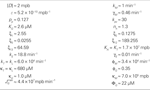Table 2. Parameter values for the lac operon model. These parameters were taken from Santillán (2008), except for KA y KT, which were re-estimated in this work. Here, mpb stands for “molecules per average-size bacterium.”

### Bifurcation Diagram Calculation

The first step to calculate bifurcation diagrams is to identify the system steady states. Given the model complexity it was not possible to get an analytic expression whose roots rendered the steady states. Thus we proceeded as follows:

1. Given the values of [Ge] and [Te] we solved numerically the model equations (using algorithm lsode of Octave) with [Le] = 0 μM and the following initial conditions: [M] = 0 mpb, [E] = 0 mpb, [L] = 0 mpb and [T] = 0 mpb. These initial conditions correspond to an state where all molecule counts are smaller than those in any uninduced operon state. Here and thereafter mpb denotes “molecules per average-size bacterium.” We carried out the simulations for 2,000 min to ensure that the system reaches an steady state.

2. We solved the model equations again, taking the same [Ge], [Te], and [Le] values, but considering the following initial conditions: [M] = 6 mpb, [E] = 1000 mpb, [L] = 1 × 108 mpb, and [T] = 1 × 108 mpb. These initial conditions correspond to an state where all molecule counts are larger than those in any induced operon state. Once more, the simulations were carried out for 2,000 min.

3. If the system is monostable for the given [Ge], [Te], and [Le] values, the steady state solutions calculated in the previous steps shall be the same, otherwise the system is bistable. In particular, we considered that the system is monostable whenever the difference between the stationary enzyme counts resulting from the simulations in steps 1 and 2 (ΔE) is smaller than 10−2 mpb.

4. Steps 1, 2, and 3 were repeated for many increasing [Le] values to determine the upper and lower borders of the bistability region in the [Le] vs. [Ge] parameter space.

5. Steps 1–4 were repeated for many increasing [Ge] values and the bifurcation diagram was drawn for the corresponding [Te] value.

6. Finally, steps 1–5 were repeated for different [Te] values and the corresponding bifurcation diagrams were drawn.

This method detects bistability whenever it exists for the following reasons. If the system is monostable, it will converge to the only available steady state regardless of the initial condition. However, if the system is bistable, each of the available stable steady states will have its own basin of attraction. Therefore, if we pick two initial conditions, one in each basin, and solve the model equations, the system will evolve to two different steady states. Finally, since the molecule count of all chemical species in one (the other) of the chosen initial states is smaller (larger) than that of the uninduced (induced) steady state, we can assure that these initial conditions lie in different basins.

The method accuracy depends on the chosen ΔE threshold and on the total simulation time; the smaller the threshold and the longer the simulation time, the more accurate the method. We carried out a numerical convergence analysis in a few selected cases and found that decreasing the threshold and increasing the simulation time beyond the reported values does not change the obtained bifurcation diagrams.

### Stochastic Simulations

To carry out stochastic simulations of the lac operon dynamics we employed a variation of the Gillespie algorithm introduced by Cao et al. (2005), which we implemented in Octave. The algorithm proceeds as follows. First, we assumed that transcription, mRNA degradation, translation, and enzyme degradation are slow reactions as compared with all the other reactions in the operon regulatory pathway. The stoichiometry of these reactions is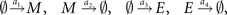while the reaction propensities are given by

α1 = kM[D]ppc([Ge])𝒫R([A],[T]),

α2 = γM + μ,

α3 = kEM,

α4 = γE + μ.

Once reaction scheme is determined, the following steps are iteratively carried out:

1. Let X = ([M],[E]) denote the system state. Initialize the simulation time (t = t0) and the state of the system (X = X0).

2. Calculate the partial equilibrium state of all fast variables (i.e., all the variables involved in fast but not in slow reactions). This is done by assuming that all fast reactions are in equilibrium given the values of [M] and [E], and by solving for the values of the fast variables.

3. Calculate the propensities of the slow reactions and their sum (a0 = a1 + a2 + a3 + a4).

4. Calculate two random numbers (r1 and r2) and calculate the time τ and the j index of the next reaction: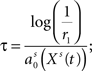j must satisfy: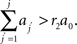5. Update the time (t = t + τ) and the system state by making the j-th reaction occur.

## Results

Following Santillán (2008), we started by simulating the experiments reported by Ozbudak et al. (2004), who quantified the lac operon bistable behavior when it is induced with TMG. To calculate the corresponding bifurcation points we set [Le] = 0 and varied [Ge] and [Te] as described in the Methods. The resulting bifurcation diagram is plotted in Figure 3A in the [Te] vs. [Ge] parameter space, together with the experimental points of Ozbudak et al. (2004). This diagram is equivalent to that in Figure 3B of Santillán (2008). The agreement between our results and those of Ozbudak et al. (2004) validate both the model and the numerical procedure to calculate the system bifurcation diagrams proposed in Section “Materials and methods.” On the other hand, observe in Figure 3A that bistability is present for all Ge values, and that both the low and high [Te] boundaries increase as [Ge] increases, reaching a plateau at about 200 μM of glucose. In particular, we have that the system becomes bistable for very low TMG levels when [Ge] < 5 μM, as reported by Ozbudak et al. (2004).

FIGURE 3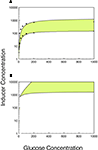Figure 3. (A) Bifurcation diagram in the [Te] vs. [Ge] parameter space, calculated for the case when the operon is induced with TMG. The black diamonds correspond to the experimental data of Ozbudak et al. (2004). (B) Bifurcation diagram in the [Le] vs. [Ge] parameter space, calculated for the case when the operon is induced with lactose. In both plots, the shaded region corresponds to bistability, while the monostable induced (top) and uninduced (bottom) regions are uncolored.

We further simulated induction of the operon with lactose, solely. To do that we set [Te] = 0 and repeated the calculations described in the previous paragraph. The resulting bifurcation diagram is plotted in Figure 3B. Note that the bistable region disappears via a cusp bifurcation for extremely low [Ge] values. On the other hand, when compared with that obtained with TMG induction, much larger [Le] values are needed to enter the bistability region. As a matter of fact, the lower [Le] bifurcation value slowly grows as [Ge] increases until about 200 μM, and reaches a plateau afterward. Conversely, the upper [Le] boundary of the bistability region increases steadily for all the analyzed [Ge] values. These results corresponds to those reported in Figure 3F of Santillán (2008).

Next, we investigated the effect of using a mixture of lactose and TMG (to induce the lac operon) on the system bistable behavior. We calculated the system bifurcation diagrams with many different values for [Le] and [Te]. To illustrate our results we show in Figure 4A the bifurcation diagram (in the [Le] vs. [Ge] parameter space) calculated with [Te] = 20 μM. Observe that, in this case, the bistability region has a peculiar shape that involves the disappearance of the cusp catastrophe. Indeed, the addition of enough TMG in the growing medium causes the opening of the cusp and the prolongation of the bistability region down to [Le] = 0 μM. An interesting consequence of this bifurcation diagram is the fact that we can now make the system switch between the uninduced and the induced steady states by keeping [Ge] constant and changing [Le] (as in the experiments by Ozbudak et al., 2004), but also by keeping [Le] constant and changing [Ge]. In particular, this last switching can be attained with very low [Le] concentrations.

FIGURE 4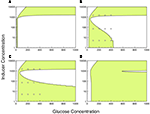Figure 4. Bifurcation diagrams in the [Le] vs. [Ge] parameter space, calculated with the present model when the lac operon is induced with a mixture of lactose and TMG. [Te] = 20 μM in (A), [Te] = 130 μM in (B), [Te] = 150 μM in (C), and [Te] = 350 μM in (D). In all cases, the shaded region correspond to the bistability region, while the monostable induced (top) and uninduced (bottom) regions are uncolored. The open circles in graphs (B,C) denote the [Ge] and [Le] values for which the stochastic simulations in Figures 5 and 6 were carried out. The stair-like appearance of the borderlines is due to the resolution of our numerical calculations.

We also calculated the system bifurcation diagram when [Te] = 130, 150, 350 μM. The results are shown in Figures 4B–D. These diagrams are qualitatively similar to that in Figure 4A in the sense that the cusp catastrophe disappears prolonging the bistable region down to [Le] = 0. However, the bistability region for [Le] < 100 μM gets wider as [Te] increases. These results are in agreement with those of Narang and Pilyugin (2008), who studied induction of the lac operon when the bacterial population grow in a mixture of lactose and glucose, and concluded that the lac operon is much more prone to bistability if the medium contains carbon sources that cannot be metabolized by the lac enzymes.

To account for the intrinsic noise caused by the stochastic timing of transcription, translation, and mRNA and enzyme degradation, we carried out stochastic simulations of the operon dynamics as described in Section “Materials and Methods.” First, we set [Te] = 130 μM, as in Figure 4B, and performed simulations for the [Ge] and [Le] values corresponding to the 12 different points shown there. For each simulation we calculated the histogram of the number of permease molecules and the results are shown in Figure 5. In all cases, we took four million simulation steps to guaranty that the system switches several times between the uninduced and the induced states, whenever the ([Ge],[Le]) point lies inside the bistability region. In fact, we chose such simulation length after performing a numerical convergence analysis and noticing that the shape of all histograms does not change after one million steps.

FIGURE 5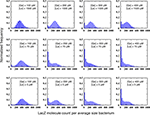Figure 5 . Histograms of LacZ polypeptide molecule count per bacterium, calculated from stochastic simulations carried out with the set ([Ge], [Le]) points shown in Figure 4B and [Te] = 130 μM.

We repeated the simulations described in the previous paragraph for [Te] = 150 μM, as in Figure 4C. One simulation was performed for each of the ([Ge],[Le]) points shown there. The histograms of the number of permease molecules, calculated for each simulation, are shown in Figure 6.

FIGURE 6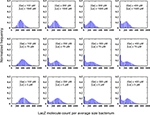Figure 6. Histograms of LacZ polypeptide molecule count per bacterium, calculated from stochastic simulations carried out with the set ([Ge],[Le]) points shown in Figure 4C and [Te] = 150 μM.

We can see in Figures 5 and 6 that, as expected, all the histograms corresponding to ([Ge], [Le]) points lying outside of bistability regions are monomodal. On the other hand, not all the histograms corresponding to ([Ge], [Le]) points within the bistability region are clearly bimodal. In some cases the histogram bimodality is blurred by the wide dispersion of one or the two modes. Finally, we observe that all histograms corresponding to bistable points located close to the uninduced monostable region have a distinct bimodal shape. This suggests that the uninduced steady state is more strongly stable in all cases.

## Discussion and Conclusions

The current understanding of the lac operon invokes bistability to explain how E. coli consumes glucose and lactose in the most efficient possible way (Lehninger et al., 2005). Namely, the genes necessary to uptake and metabolize lactose are only turned on when this sugar is present (at high levels) in the growing environment, while glucose (the bacterium favorite carbon source) is absent. Nonetheless, recent experimental and modeling works have open a controversy regarding the existence of bistability in this system under natural conditions. While van Hoek and Hogeweg (2006) propose that the wild-type lac operon is not a bistable switch when the bacterial population grows in a lactose rich medium for several generations, Santillán (2008) argue that bistability is present under such conditions and plays an important evolutionary role.

In order to contribute to the clarification of the above referred controversy, we have extended the model developed by Santillán (2008) for the lactose operon in E. coli to account for the presence of both lactose and TMG in the bacterial growing medium. Our aim is to make quantitative predictions regarding the bistable behavior of this system, which can be experimentally tested to validate the model itself. We have paid special attention to the estimation of all the model parameters from reported experimental data.

To test the extended model we reproduced the bifurcation diagrams calculated by Santillán (2008) for when the lactose operon is induced with either TMG or lactose. Our results are completely equivalent to those of Santillán (2008), as one can verify by comparing the bifurcation diagrams in Figures 3A,B with the corresponding diagrams in Santillán (2008).

Afterward, we investigated the effect of inducing the lac operon of E. coli with a mixture of lactose and TMG. We did this by using both a deterministic model and stochastic simulations. The results are summarized in Figures 46. When comparing with the bistability diagram corresponding to induction by lactose – Figure 3B, we can see that the addition of enough TMG makes the cusp catastrophe disappear and prolongs the bistability region down to 0 in the [Le] axis – see Figures 4A–D. These results were corroborated by means of stochastic simulations – see Figures 5 and 6.

The results described in the previous paragraph predict that bistability can be found at much lower [Le] values when a mixture of lactose and TMG is employed, as compared with the situation where lactose is the only inducer. Moreover, the novel shape of the bistability region also implies that bistability could be experimentally tested with the setup of Ozbudak et al. (2004) by fixing the extracellular glucose and TMG concentrations in the growing medium and varying the level of lactose, but also by fixing the extracellular lactose and TMG levels and varying the glucose concentration. If validated experimentally, these predictions would speak in favor of the Santillán (2008) model, as well as of his conclusions regarding bistability in the lac operon of E. coli. The fact that some bistable points, according to the deterministic model, are hard to identify through stochastic simulations does not invalidate the previously proposed experiments: smaller bistability regions would be found than those predicted by the deterministic model, but they could certainly be recognized.

The importance of unveiling whether the lac operon of E. coli is bistable or not under natural conditions transcends this system. The reason is that the gene networks responsible for the alternate consumption of glucose and tryptophan in E. coli (Konan and Yanofsky, 1997), and of glucose and galactose in S. cerevisiae (Acar et al., 2005; Bhat and Iyer, 2009), have the same architecture as the lac operon of E. coli. That is, the regulatory pathway controlling the expression of the genes involved in the transport and metabolism of carbon sources other than glucose seems to be conserved over different metabolic networks and over different species. Thus, a complete understanding of the lac operon dynamics may help to understand the performance of a whole class of gene networks. Finally, experiments similar to the ones here proposed may help to elucidate the existence of bistability in other gene networks with the same architecture.

## Appendix

### Model Development

#### Interaction of the lac repressor with a single operator

The lac repressor is a homo-tetramer made up of two identical functional dimers. Each monomer in the dimer can be bound by either an allolactose (A) or a TMG (T) molecule. When all the monomers in the repressor are free, each dimer can bind an operator and so the repressor can bind two operators simultaneously. When one or the two monomers in one dimer of the repressor are bound by either A or T, and the opposite dimer is free, the repressor can still bind an operator. However, when two or more monomers in opposite sides are bound by A or T, the repressor cannot bind any operator.

In what follows R represents a repressor completely free from allolactose and TMG, RA (RT) denote a repressor bound by a single allolactose (TMG) molecule, R2A (R2T) indicates a repressor bound by two allolactose (TMG) molecules, and finally RAT corresponds to a repressor bound by one allolactose and one TMG molecule. We followed Santillán (2008) to found the fractional concentrations of all these complexes. That is, we wrote down all the chemical equations through which these complexes are formed, as well as the equilibrium equation for each one of them. Afterward, we wrote conservation equation for the total repressor concentration. And, finally, we solve for the concentrations of all the possible repressor complexes. The results are: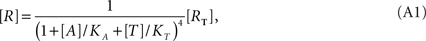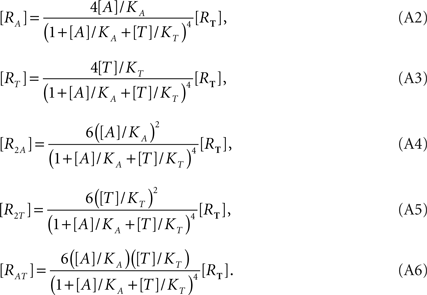#### DNA folding

There are three different operators in the lactose operon. A repressor molecule with at least one free dimer can bind any one of these operators. Furthermore, DNA can fold in such a way that a free repressor molecule can bind two different operators simultaneously. In what follows O denotes the state where all three operators are free; O3R corresponds to the state where all operators are bound by different operators; the state where only the i-th operators is bound by a repressor is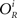;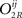indicates the state where operators i and j are bound by different repressors;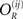represents the state where operators i and j are both bound by a single repressor; and finally,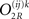corresponds to the state where operators i and j are bound by the same repressor, while operator k is bound by another repressor.

Santillán (2008) deduced expressions for the probability of all possible operator states when the lac operon is induced solely with lactose. Following an analogous procedure we found the probabilities for all the operator states, taking into consideration that each binding site in a repressor molecule can be bound by either an allolactose or a TMG molecule. In the forthcoming paragraph we present the results, but omit the algebraic procedure for the sake of brevity.

The fraction of free operator concentration as a function of [R] and [R′] is: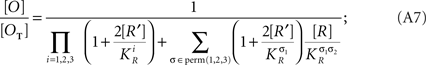the fraction of operators in stateis: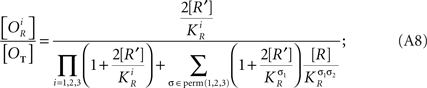the probability that the operator complex is in stateis: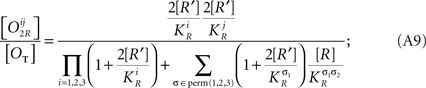the probability that all three operators are bound by different repressors is: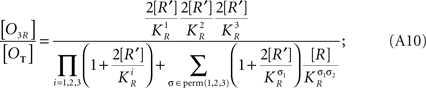the probability of stateis: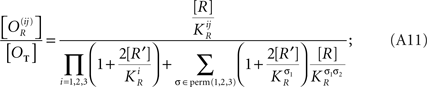and finally, the fraction of operators in stateis: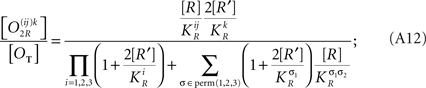In the above equations, [R] is given by (A1) while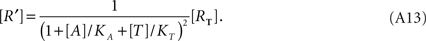#### Transcription initiation and mRNA dynamics

Following Santillán (2008), transcription can only start if operator O1 is free. That is, a polymerase can start transcription in the following operator binding states: O,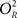,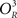,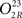, and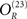.

Let [D] denote the concentration of operon copies, and kM the maximum transcription initiation rate of promoter P. From the discussion in the previous paragraph and the results of the previous subsection, the rate of transcription initiation comes out to be: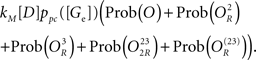Then, the transcription initiation rate at the lac promoter is:

kM[D]ppc([Ge]) 𝒫R([A],[T]),

where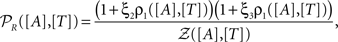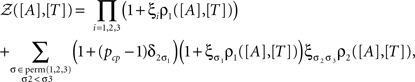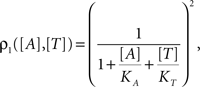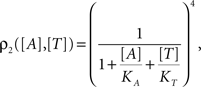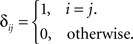and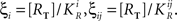Let μ and γ respectively denote the bacterial growth rate and the mRNA degradation rate. From this, the differential equation governing the mRNA (M) dynamics is: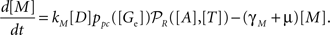𝒫R([A],[T]) accounts for the cooperativity in the lactose operon of E. coli. Here we employ the expression derived by Santillán (2008) for this function.

#### Lactose, allolactose, and TMG dynamics

Let [Ge], [Le], [Te], [L], [A], and [T] respectively represent the concentrations of external glucose, external lactose, external TMG, intracellular lactose, intracellular allolactose, and intracellular TMG. Following Santillán (2008), the efficiencies of β-permease to transport lactose and TMG respectively are written as: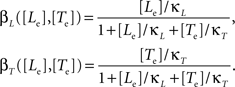Lactose and TMG transport by β-permease is inhibited by external glucose (a phenomenon know as inducer exclusion). The decrease on the uptake rate as a function of [Ge] can be modeled by (Ozbudak et al., 2004):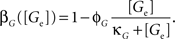Let kL and kT be the maximum rates of lactose and TMG uptake, respectively. Then, the corresponding uptake rates are:

kLβL([Le],[Te])βG([Ge])[Q], and kTβT([Le],[Te])βG([Ge])[Q].

Once lactose is internalized, it is metabolized by β-galactosidase. Approximately half of the lactose molecules are directly converted into glucose and galactose, while the rest are turned into allolactose. Allolactose is later converted into glucose and galactose by the same enzyme (β-galactosidase). Following Santillán (2008), and taking into account the considerations above, the dynamics of intracellular lactose can be modeled by the following equation:where ℳ ([L]) = [L]/(κM + [L]) is the rate or lactose metabolism into glucose and galactose, and the factor 2 accounts for the fact that lactose is metabolized also into allolactose at approximately the same rate. We can also assume according to Santillán (2008) that

[A] = [L].

Similarly, the equation governing the dynamics of intracellular TMG results to be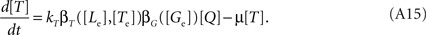Since TMG is not metabolized by β-galactosidase, the corresponding term is absent.

### Parameter Estimation

Given that the present model is a generalization of that introduced by Santillán (2008), we took most of the parameter values estimated in such reference. It must be emphasized that such parameters values were all estimated from data reported for E. coli.

Nonetheless, we found more accurate experimental dates we regarding the maximum initiation transcription rate, kM, and thus we re-estimated this parameter as follows. Russell and Bennett (1982) report that the trp promoter is approximately five-fold stronger than the lac promoter. On the other hand, according to Santillán and Zerón (2004) the maximum initiation transcription rate of the trp operón is 5.1 min−1. From this data we estimate

kM = 1.0 min−1.

Having a new value for kM obligates us to re-estimate parameter KT by fitting the bifurcation diagram predicted by our model, with only TMG like inductor, to the experimental results of Ozbudak et al. (2004). The value we obtained through this procedure is

KT = 1.7 × 107 mpb.

On the other hand, since we are considering both lactose and TMG, there are parameters in this model which do no appear in the model by Santillán (2008). These parameters are the affinity of lactose to the repressor (KA), the affinity of TMG to the lac permease (κT), and the maximum rates of TMG and lactose uptake per permease (kT). Given that TMG is a chemical analogue of lactose, we simply assumed that KA = KT, κA = κT, and kA = kT.

## Conflict of Interest Statement

The authors declare that the research was conducted in the absence of any commercial or financial relationships that could be construed as a potential conflict of interest.

## Acknowledgments

The authors are thankful to Eduardo S. Zeron for his fruitful advice. This research was partially supported by Consejo Nacional de Ciencia y Tecnología (CONACyT, Mexico) under grant 55228.

## References

Acar, M., Becskei, A., and van Oudenaarden, A. (2005). Enhancement of cellular memory by reducing stochastic transitions. Nature 435, 228–232.

Angeli, D., Ferrell, J. E. Jr., and Sontag, E. D. (2004). Detection of multistability, bifurcations, and hysteresis in a large class of biological positive-feedback systems. Proc. Natl. Acad. Sci. U.S.A. 101, 1822–1827.

Bagowski, C. P., Besser, J., Frey, C. R., and Ferrell, J. E. Jr. (2003). The JNK cascade as a biochemical switch in mammalian cells: ultrasensitive and all-or-none responses. Curr. Biol. 13, 315–320.

Bagowski, C. P., and Ferrell, J. E. (2001). Bistability in the JNK cascade. Curr. Biol. 11, 1176–1182.

Bhalla, U. S., Ram, P. T., and Iyengar, R. (2002). MAP kinase phosphatase as a locus of flexibility in a mitogen-activated protein kinase signaling network. Science 297, 1018–1023.

Bhat, P. J., and Iyer, R. S. (2009). Epigenetics of the yeast galactose genetic switch. J. Biosci. 34, 513–522.

Cao, Y., Gillespie, D. T., and Petzold, L. R. (2005). Multiscale stochastic simulation algorithm with stochastic partial equilibrium assumption for chemically reacting systems. J. Comput. Biol. 206, 395–411.

Chung, J. D., and Stephanopoulos, G. (1996). On physiological multiplicity and population heterogeneity of biological systems. Chem. Eng. Sci. 51, 1509–1521.

CrossRef Full Text

Cohn, M., and Horibata, K. (1959). Analysis of the differentiation and of the heterogeneity within a population of Escherichia coli undergoing induced beta-galactosidase synthesis. J. Bacteriol. 78, 613–623.

Cross, F. R., Archambault, V., Miller, M., and Klovstad, M. (2002). Testing a mathematical model of the yeast cell cycle. Mol. Biol. Cell 13, 52–70.

Ferrell, J. E. (2002). Self-perpetuating states in signal transduction: positive feedback, double-negative feedback, and bistability. Curr. Opin. Chem. Biol. 6, 140–148.

Ferrell, J. E., Jr., and Machleder, E. M. (1998). The biochemical basis of an all-or-none cell fate switch in Xenopus oocytes. Science 280, 895–898.

Konan, K. V., and Yanofsky, C. (1997). Regulation of the Escherichia coli tna operon: nascent leader peptide control at the tnaC stop codon. J. Bacteriol. 179, 1774–1779.

Lehninger, A. L., Nelson, D. L., and Cox, M. M. (2005). Lehninger Principles of Biochemistry, 4th Edn. New York: W.H. Freeman. http://www.loc.gov/catdir/enhancements/fy0912/200410171%6-d.html

Lewis, M. (2005). The lac repressor. C. R. Biol. 328, 521–548.

Monod, J. (1941). Recherches sur la croissance des cultures bactériennes. Ph.D. thesis, Université de Paris, Paris.

Narang, A. (2007). Effect of DNA looping on the induction kinetics of the lac operon. J. Theor. Biol. 247, 695–712.

Narang, A., and Pilyugin, S. S. (2008). Bistability of the lac operon during growth of Escherichia coli on lactose and lactose + glucose. Bull. Math. Biol. 70, 1032–1064.

Novick, A., and Weiner, M. (1957). Enzyme induction as an all-or-none phenomenon. Proc. Natl. Acad. Sci. U.S.A. 43, 553–566.

Oehler, S., Eismann, E. R., Krämer, H., and Müller-Hill, B. (1990). The three operators of the lac operon cooperate in repression. EMBO J. 9, 973–979.

Ozbudak, E. M., Thattai, M., Lim, H. N., Shraiman, B. I., and Van Oudenaarden, A. (2004). Multistability in the lactose utilization network of Escherichia coli. Nature 427, 737–740.

Pomerening, J. R., Sontag, E. D., and Ferrell, J. E. Jr. (2003). Building a cell cycle oscillator: hysteresis and bistability in the activation of Cdc2. Nat. Cell Biol. 5, 346–351.

Reznikoff, W. S. (1992). The lactose operon-controlling elements: a complex paradigm. Mol. Microbiol. 6, 2419–2422.

Rietkerk, M., Dekker, S. F., de Ruiter, P. C., and van de Koppel, J. (2004). Self-organized patchiness and catastrophic shifts in ecosystems. Science 305, 1926–1929.

Russell, D. R., and Bennett, G. N. (1982). Construction and analysis of in vivo activity of E. coli promoter hybrids and promoter mutants that alter the −35 to −10 spacing. Gene 20, 231–243.

Santillán, M. (2008). Bistable behavior in a model of the lac operon in Escherichia coli with variable growth rate. Biophys. J. 94, 2065–2081.

Santillán, M., Mackey, M. C., and Zeron, E. S. (2007). Origin of bistability in the lac operon. Biophys. J. 92, 3830–3842.

Santillán, M., and Zerón, E. S. (2004). Dynamic influence of feedback enzyme inhibition and transcription attenuation on the tryptophan operon response to nutritional shifts. J. Theor. Biol. 231, 287–298.

Sha, W., Moore, J., Chen, K., Lassaletta, A. D., Yi, C. S., Tyson, J. J., and Sible, J. C. (2003). Hysteresis drives cell-cycle transitions in Xenopus laevis egg extracts. Proc. Natl. Acad. Sci. U.S.A. 100, 975–980.

van Hoek, M. J. A., and Hogeweg, P. (2006). In silico evolved lac operons exhibit bistability for artificial inducers, but not for lactose. Biophys. J. 91, 2833–2843.

Wilson, C. J., Zhan, H., Swint-Kruse, L., and Matthews, K. S. (2007). The lactose repressor system, paradigms for regulation, allosteric behavior and protein folding. Cell. Mol. Life Sci. 64, 3–16.

Keywords: systems biology, computational biology, non-linear dynamics

Citation: Díaz-Hernández O and Santillán M (2010) Bistable behavior of the lac operon in E. coli when induced with a mixture of lactose and TMG. Front. Physiol 1:22. doi: 10.3389/fphys.2010.00022

Received: 16 February 2010; Paper pending published: 12 March 2010;
Accepted: 22 June 2010; Published online: 19 July 2010

Edited by:

Raina Robeva, Sweet Briar College, USA

Reviewed by:

Julio Vera González, University of Rostock, Germany
Jongrae Kim, University of Glasgow, UK
William Mather, University of California San Diego, USA

Copyright: © 2010 Díaz-Hernández and Santillán. This is an open-access article subject to an exclusive license agreement between the authors and the Frontiers Research Foundation, which permits unrestricted use, distribution, and reproduction in any medium, provided the original authors and source are credited.

*Correspondence: Moisés Santillán, Unidad Monterrey, Centro de Investigación y de Estudios Avanzados del IPN, Vìa del Conocimiento 201, Parque de Investigación e Innovación Tecnológica, 66600 Apodaca NL, Mexico. e-mail: msantillan@cinvestav.mx